# Chocolate chip

Out of 28 cookies, 3/4 are chocolate chip. How many cookies are chocolate chip?

x =  21

### Step-by-step explanation: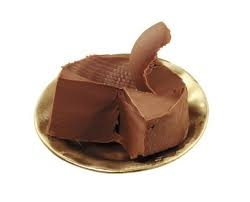Did you find an error or inaccuracy? Feel free to write us. Thank you!Tips to related online calculators
Need help to calculate sum, simplify or multiply fractions? Try our fraction calculator.

## Related math problems and questions: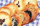In a cookie jar, 1/4 of the cookies are chocolate chip and 1/2 of the rest are peanut butter. What fraction of all the cookies are peanut butter?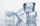Miriam is making 3 1/2 dozen cookies. If 2/7 of the cookies have icing, how many dozen cookies have icing?
• How manyHow many integers are greater than 547/3 and less than 931/4?
• FlourKim needs 3/4 cup of flour to make 12 cookies. How much flour would she need to make 60 cookies?
• Fractions and mixed numerals(a) Convert the following mixed numbers to improper fractions. i. 3 5/8 ii. 7 7/6 (b) Convert the following improper fraction to mixed number. i. 13/4 ii. 78/5 (c) Simplify these fractions to their lowest terms. i. 36/42 ii. 27/45 2. evaluate following ex
• CompareCompare fractions (34)/(3) and (12)/(4). Which fraction of the lower?
• Multiplication negativesJosh says that (4)(-7)(-1) is equal to (4)(7). Evangelina says (4)(-7)(-1) is equal to (-28)(-1) . Who is correct? Explain your reasoning.
• Simplest form of a fractionWhich one of the following fraction after reducing in simplest form is not equal to 3/2? a) 15/20 b) 12/8 c) 27/18 d) 6/4
• Hours 3How many hours are in 3 2/3 days?
• Hotel 4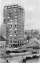A 360 room hotel has 1/3 of its rooms occupied at present . How many rooms are empty?
• Find the 28Find the area of a square with side length 4/5 yard.
• Quotient 3If the quotient of 8/13 and 2 is subtracted from the product of 1 3/4 and 8/21, what is the difference?
• Golf ballsOf the 28 golf balls, 1/7 are yellow. How many golf balls are yellow? Use the model to help you. Enter your answer in the box.
• Numbers divisionWith what number should be divided mixed number 2 3/4 to get 11/12?
• Equivalent fractions 2Write the equivalent multiplication expression. 2 1/6÷3/4
• Change the numbers in the ratio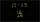Change the numbers 29, 38, and 43 in a 3: 4 ratio.
• Sort fractionsWhich is larger 3/7, 3/8, 3/9, 3/6 =